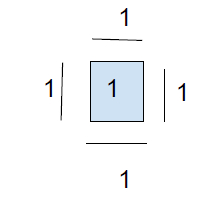# Find perimeter of shapes formed with 1s in binary matrix in C++

C++Server Side ProgrammingProgramming

#### C in Depth: The Complete C Programming Guide for Beginners

45 Lectures 4.5 hours

#### Practical C++: Learn C++ Basics Step by Step

Most Popular

50 Lectures 4.5 hours

#### Master C and Embedded C Programming- Learn as you go

66 Lectures 5.5 hours

In this problem, we are given a binary matrix bin[][] of size nXm consisting of 0’s and 1’s only. Our task is to Find perimeter of shapes formed with 1s in a binary matrix.

The perimeter taken will cover the figure from all sides, i.e.

For 1 single value, the perimeter is 4.Let’s take an example to understand the problem,

## Input

bin[][] = [1, 0]
[1, 0]

## Output

6

## Explanation

The cells (0,0) and (1, 0) are connected making a rectangle of sides 2 and 1. The perimeter is 6.

## Solution Approach

A simple solution to the problem is simply finding all one and their contributions to the perimeter and then add all to find the value.

The contribution of a 1 to the perimeter in matrix is, The maximum contribution is 4, when the 1 alone contributes to the perimeter

The minimum contribution is 0, when the 1 is surrounded by 1’s by all sides.

So, for each element of the matrix, we need to check if it is 1 or not. For all 1’s, we will find its neighbours and then contribution to the perimeter and then finally the perimeter.

Program to illustrate the working of our solution,

## Example

Live Demo

#include<iostream>
using namespace std;
#define R 3
#define C 5
int contibutionToPerimeter(int mat[][C], int i, int j) {
int neighbours = 0;
if (i > 0 && mat[i - 1][j])
neighbours++;
if (j > 0 && mat[i][j - 1])
neighbours++;
if (i < R-1 && mat[i + 1][j])
neighbours++;
if (j < C-1 && mat[i][j + 1])
neighbours++;
return (4 - neighbours);
}
int calcPerimeter(int mat[R][C]){
int perimeter = 0;
for (int i = 0; i < R; i++)
for (int j = 0; j < C; j++)
if (mat[i][j] == 1)
perimeter += contibutionToPerimeter(mat, i ,j);
return perimeter;
}
int main() {
int mat[R][C] = { {0, 1, 0, 0, 0},
{1, 1, 1, 1, 0},
{1, 1, 0, 1, 1} };
cout<<"The perimeter of shapes from formed with 1s is "<<calcPerimeter(mat);
return 0;
}

## Output

The perimeter of shapes from formed with 1s is 18